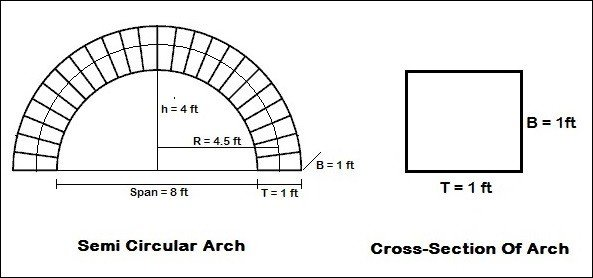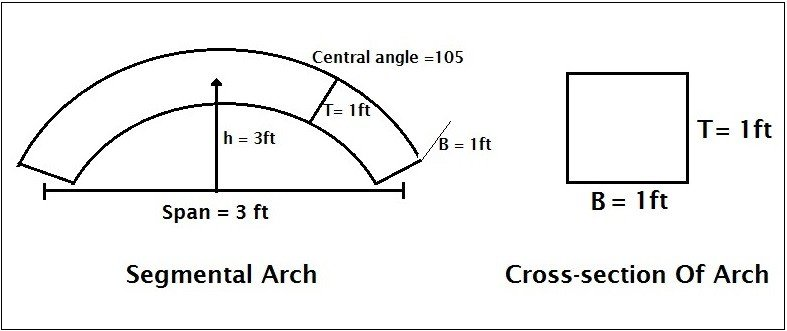# How To Calculate Quantity Of Materials In Arch

In this article, I will discuss how two calculate quantity of materials in arch.

Contents

## 1. Semi Circular Arch:

Given:

Span = 8 ft

Height = 4 ft

Thickness = 1 ftTo calculate the quantity of materials in semi circular arch we need to calculate the volume of arch.

Volume of arch = Cross-section of arch x Arch length

We know Cross-section area = Thickness of arch x Breadth of arch = T x B

From the above image, you can see the thickness and breadth are 1 ft.

Cross-section of arch = 1 x 1 = 1 ft²

Now Arch length = Circumference of half circle (The arch is semi circular)

We know circumference of circle = 2πR

Circumference of half circle = 2πR/2 = πR

We don’t what is R. So we need to find out the ‘R’.

R = Half span + Thickness/2 = 4 +1/2 = 4.5 ft.

Arch length = πR =3.14 x 4.5 = 14.13 ft.

Quantity of materials in arch = 1 x 14.13 = 14.13 cft.

## 2. Segmental Arch:

Given:

Span = 8 ft

Height = 2ft

Thickness = 1 ft

Central angle = 105°## Solution:

Volume arch = Cross-section of arch x Arch length

We know Cross-section area = Thickness of arch x Breadth of arch = T x B

Arch length = θπR/180

Now R = (a² + h²)/2h     [a =half span = 8/2 =4]

=(4² + 3²)/2 x 3 = 25/6 =4.16 ft.

Arch length = (105 x 3.14 x 4.16)/180 = 7.61

Quantity of materials in arch = 1 x 7.61 = 7.61 cft.

### 2 thoughts on “How To Calculate Quantity Of Materials In Arch”

1. Plz send Me all formula for civil and tables for how to calculate basic knowledge